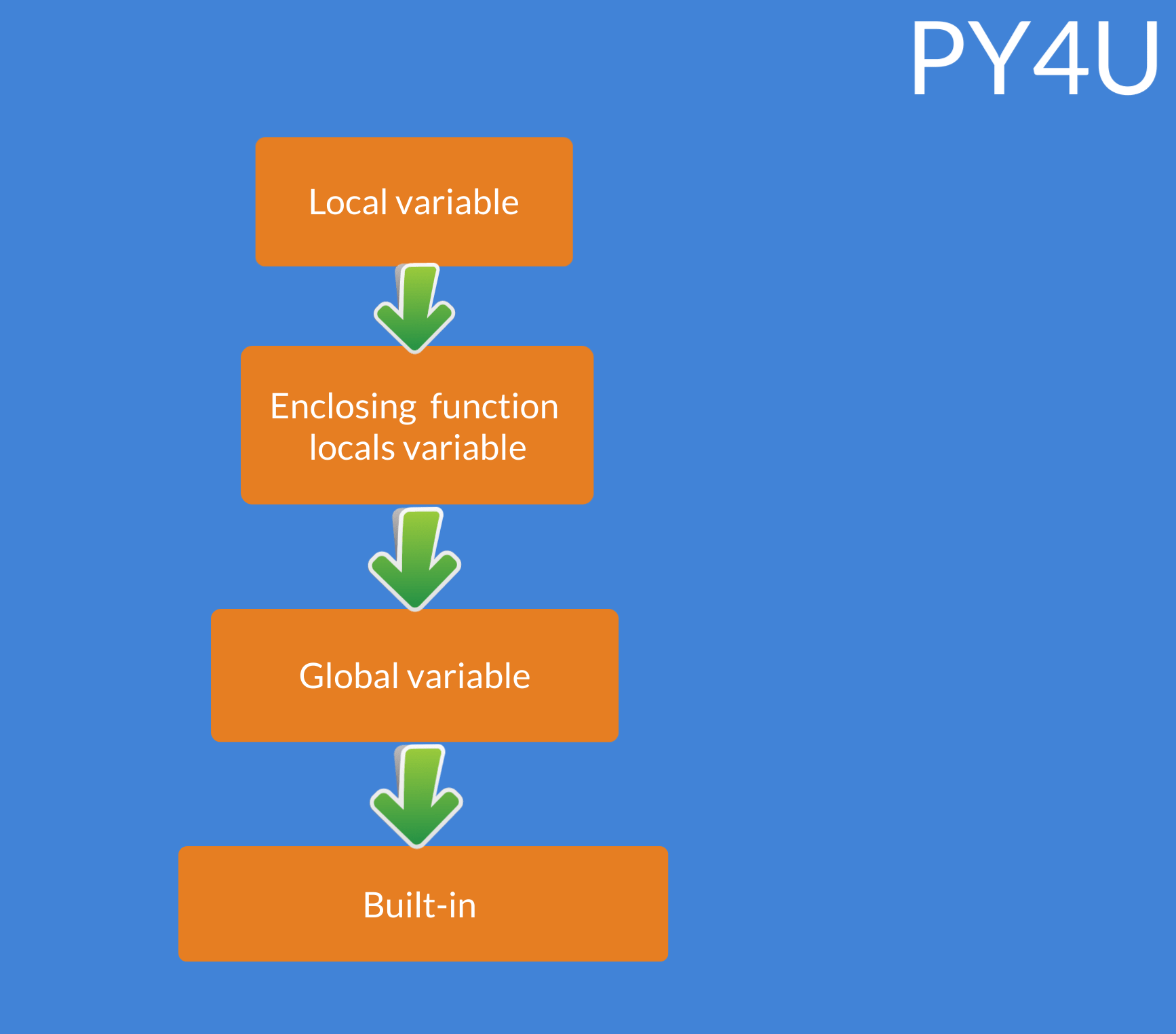Scope Of A Variable

The scope of a variable determines the visibility of the variable to other parts of the code. While dealing with functions , the scope of a variable plays an very important role . Let us understand this with help of an example.

What do you think should be the output of the code given below?

x = 100
def scope():
x=50 # value of x reassigned

scope()  # scope function invoked
print(x)
output:
100

Did you expect the output to be 50 or 100 ??. We will answer all the questions by learning the LEGB rule that Python follows for deciding the scope of a variable.

LEGB Rule

L : Local

Variables declared within a function ( def or lambda ) that are local ( in terms of scope ) to that function .So in the code given below , x is locally defined for the local() function and hence its scope is limited within the function only.

def local():
x=15
return x
E : Enclosing function locals

If there is no local variable inside a function , then Python looks for variables that are local to the enclosing function . So in the code given below , x has enclosing function locals scope with respect to function b ( and local to function a ). And that is we get 15 as the output.

def a():
x = 15
def b():
# no local declaration
print(x)
b()

a()
output:
15
G : Global

if there is no local and enclosing function locals variable , then Python looks for global variables. So in the code given below , variable x is a global variable .

x=15
def a():
#no local declaration
def b():
# no local declaration
print(x)
b()

a()
output:
15
B : Built-in

If Python cannot find a specific variable declaration in the whole code then it starts to look in the built-in modules. So when you are using functions like range , len ... well Python goes looking in the built-in modules to find their definition. The infographic below explains the chain that python follows to work with variables.Now coming back to the problem we discussed at the top of this section

x = 100
def scope():
x=50 # value of x reassigned

scope()  # scope function invoked
print(x)

The variable x that is defined within the scope() function is local to that function and so therefore it has no impact on the globally declared x variable and so that is why we get 100 as our output and not 50.

Popular Tutorials